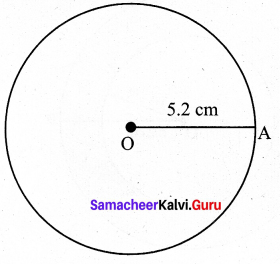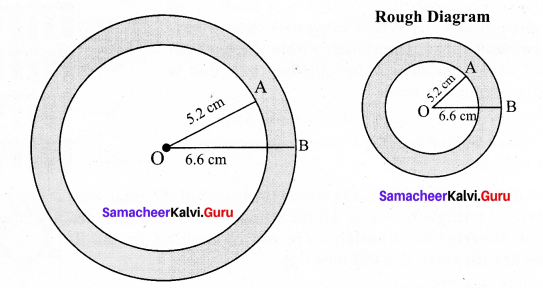Samacheer Kalvi 7th Maths Solutions Term 3 Chapter 4 Geometry Additional Questions

Students can Download Maths Chapter 4 Geometry Additional Questions and Answers, Notes Pdf, Samacheer Kalvi 7th Maths Book Solutions Guide Pdf helps you to revise the complete Tamilnadu State Board New Syllabus and score more marks in your examinations.

Tamilnadu Samacheer Kalvi 7th Maths Solutions Term 3 Chapter 4 Geometry Additional Questions

Exercise 4.1

Question 1.
Give some examples of shapes with no line of symmetry.
Solution:
1. A scalene Triangle
2. The letter F

Question 2.
What is the order of rotational symmetry of the letter N?
Solution:
2Exercise 4.2

Question 1.
Construct a circle of radius 5.2 cm with center ‘O’.Solution:
Step 1: Market a point‘O’on the paper.
Step 2: Extended the compass distance equal to radius 5.2 cm.
Step 3: At center ‘O’, held the compass firmly and placed the pointed end of the compass.
Step 4: Slowly rotated the compass around to get the circle.Question 2.
Draw concentric circles with radii 5.2cm and 6.6cm. Find the width of the circular ring.
Solution:Step 1: Drawn a rough diagram and marked the given measurements Taken any
Step 2: Take any point O and marked it as the center.
Step 3: With O as center, drawn a circle of radius OA = 5.2 cm.
Step 4:With O as center, drawn a circle of radius OB = 6.6 cm. Thus the concentric circles C1 and C2 are drawn.
Width of the circular ring = OB – OA = 6.6 – 5.2 = 1.4 cm